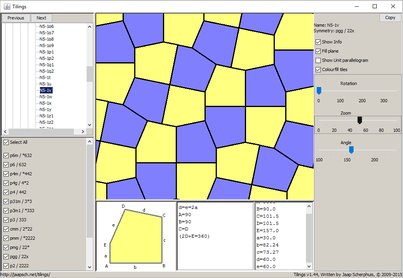Non-convex Tiles

There not as much research into tilings with non-convex tiles than with convex tiles. On this page I show some of the ones I found during my research into tiling patterns.

Non-Convex Tiles

Isohedral tilings

All triangles are convex, so for non-convex tiles we need at least four sides to each tile.

Many of the isohedral tilings with convex quadrangles have enough degrees of freedom to allow the tiles to be non-convex too, but there are no isohedral tilings that are specific to non-convex quadrangles.

Non-Convex Pentagons

There are several isohedral tilings with pentagon tiles where the tiling forces the pentagon to be non-convex, all of which are in the Tiling Viewer applet.

Non-convex Hexagons

There are many isohedral tilings specific to non-convex hexagons, but I have not (yet) implemented all of them in the Tiling Viewer applet.

2-Isohedral tilings

I found no 2-isohedral tilings that are specific to non-convex quadrangles.

Non-Convex Pentagons

There were many 2-isohedral tilings with non-convex pentagons, all of which have now been included in the Tiling Viewer applet.

Non-convex Hexagons

My program has generated all edge-to-edge tilings of non-convex hexagons, and I have implemented many but not yet all of them in the Tiling Viewer applet. I have not looked for tilings that are not edge-to-edge.

3-Isohedral tilings

Non-convex Hexagons

I have only done a few very small searches for these tilings (and only for edge-to-edge ones) so much of the search space is left unexplored. Many tiling patterns were found, but most are variations of 2-isohedral ones. A few of the more interesting patterns are shown here:

k-Isohedral tilings, k>3

I have not been able to search for these specifically, but a few interesting tilings with a particular non-convex pentagon are already known.

Non-Convex Pentagons

Written by Jaap Scherphuis, tilings a t jaapsch d o t net.